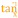# Arc Tangent

 Block Group: Trigonometric Functions Icon:The Arc Tangent block returns the arctangent, or inverse tangent, of a number, in degrees. The arctangent of n is the angle for which n is the tangent.

For information on using dataflow blocks, see Dataflow.

## Input/Output Property

The following property of the Arc Tangent block can take input and give output.

• input (number)

input defines the tangent to get the angle for.

## Output Property

The following property of the Arc Tangent block can give output but cannot take input.

• output (number)

output returns the arctangent of the input, in degrees.

## Example

The following image shows an example of the Arc Tangent block. In this example, the tangent is 1, and the arctangent is 45 degrees.